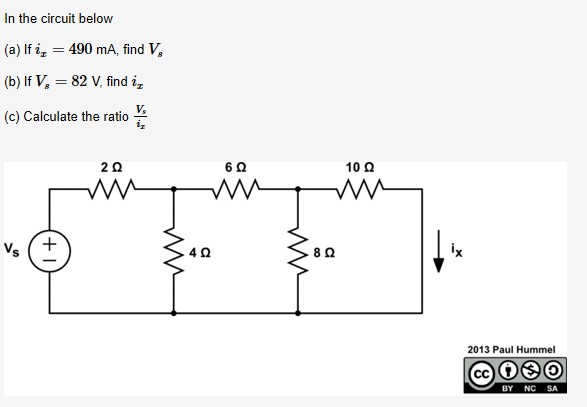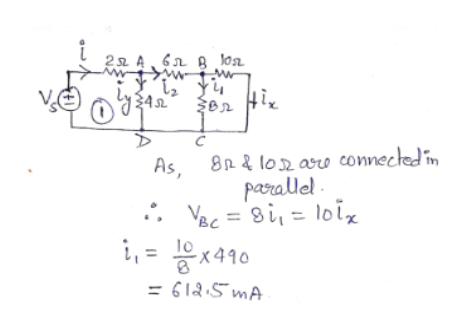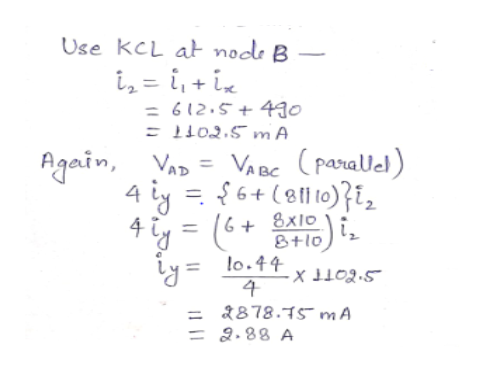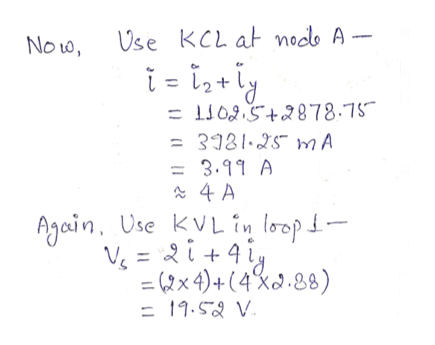# In the circuit below(a) If i, = 490 mA, find V,%3D(b) If V, = 82 V, find i,%3DV,(c) Calculate the ratio10 Qix8Q2013 Paul HummelSABYNC

Question
6 viewshelp_outlineImage TranscriptioncloseIn the circuit below (a) If i, = 490 mA, find V, %3D (b) If V, = 82 V, find i, %3D V, (c) Calculate the ratio 10 Q ix 8Q 2013 Paul Hummel SA BY NC fullscreen
check_circle

Step 1

Part (a)

The circuit is solved using Ohm’s law and Kirchhoff's voltage law and Kirchhoff's current law.

First, find each branch current and the potential drop across branches of the circuit.help_outlineImage Transcriptionclose22 4, 6n B Jo 8R & lo2 are connectedin paralle - : VBc = 8i, = loix As, %3D i, = Ex410 = 612:5 mA %3D fullscreen
Step 2

Now, use Kirchhoff’s current law to find the current in other branches of the circuit.help_outlineImage TranscriptioncloseUse kCL at node B i, = i, + iz = 612.5+490 = L102.5 m A Again, VaD = VABC (parallel) 4 iy = §6+(8ll0)}i, 4 ty = (6+ 8F10) is Ly = lo.44 %3D S'COTT - = 2878.75 mA = 2.88 A fullscreen
Step 3

Now, find the total current drawn by the circuit and use Kirc...help_outlineImage TranscriptioncloseUse KCL at nodo A - Now, i- i,+ly = L1025+2878.75 = 3981·25mA = 3.99 A Again, Use KVLin loop- V. = 2i+4iy = (2x 4)+(4%a.88) = 19.52 V. %3D fullscreen

### Want to see the full answer?

See Solution

#### Want to see this answer and more?

Solutions are written by subject experts who are available 24/7. Questions are typically answered within 1 hour.*

See Solution
*Response times may vary by subject and question.
Tagged in

### Electrical Engineering